# Langevin dynamics¶

## Introduction¶

In this tutorial, you are going to learn about Langevin dynamics, as well as two different ways to estimate the diffusion coefficient of particles in a system. Langevin dynamics is a very easy and therefore widely used technique to add Stokes friction and Brownian motion to a simulation setup.

Let's consider a single spherical colloidal particle in a fluid. Due to the absence of further particles and external fields, this particle experiences Brownian motion as a result of the interaction with the solvent molecules. While structural relaxation times for molecular fluids are of the order of $10^{-14}\,\mathrm{s}$, relevant time scales for Brownian particles are known to be in the order of $10^{-9}\,\mathrm{s}$. The distinction between slow and fast degrees of freedom allows us to describe the motion of the colloidal particle in terms of the Langevin equation. This equation of motion describes the apparent random movement of the particle in the fluid and is given by

\begin{equation} m\dot{{\bf v}}(t)=-\gamma {\bf v}(t)+{\bf f}(t), \tag{1} \end{equation}

where $m$ denotes the particle mass and ${\bf v}(t)$ its velocity. Equation (1) arises from Newton's equation of motion considering that the interaction of the spherical Brownian particle with the solvent has two contributions: 1) a friction force, which is proportional to the velocity of the particle, for not too large velocities, with proportionality constant equal to the friction constant $\gamma$; and 2) a force ${\bf f}(t)$ rapidly varying in time due to the the random collisions of the solvent molecules with the surface of the Brownian particle.

For a macroscopically large spherical particle, $\gamma$ is given by the Stokes' law

$$\gamma = 6\pi\eta_0a,$$

with $\eta_0$ the shear viscosity of the fluid and $a$ the radius of the Brownian particle. The ensemble average of the fluctuating force ${\bf f}(t)$ vanishes,

$$\langle {\bf f}(t)\rangle = 0,$$

since the systematic interaction with the fluid is made explicit in the friction term. Owing to the separation in time scales, there is no correlation between impacts in any distinct time intervals. Thus, the second moments of ${\bf f}$ satisfy

$$\langle f_i(t)f_j(t')\rangle =2\gamma k_\text{B}T \delta_{i,j}\delta(t-t'),$$

where one can see that the strength of the fluctuation force depends on the friction coefficient and the system temperature $T$. (The Boltzmann constant is denoted as $k_\text{B}$ and the two $\delta$s are Dirac delta functions with respect to particle id and time, respectively.)

The Langevin equation obviously provides a very straightforward approach to represent both Stokes friction and Brownian motion acting on a particle. However, please be aware that due to its simplicity this technique also has its limitations, i.e., the drag force and diffusion to adjacent particles are uncorrelated and hydrodynamic interactions with the particle's surroundings are neglected. Langevin dynamics should therefore be used with caution, particularly in systems with high particle densities or strong hydrodynamic coupling.

In the Langevin equation, only ensemble averaged properties of the random ${\bf f}$ are specified. Consequently, it doesn't make sense to look at a single deterministic solution of Eq. (1). Instead, one should measure ensemble averaged quantities that characterize the dynamics of the spherical Brownian particle. The simplest quantity is the so-called mean square displacement (MSD) after time $\tau$

$$\mathrm{MSD}(\tau)=\langle |{\bf r}(t+\tau)-{\bf r}(t)|^2\rangle.$$

From integration of Eq. (1) in three dimensions and considering that ${\bf v}(t)=\dot{{\bf r}}(t)$, one can obtain that

$$\mathrm{MSD}(\tau)=6D\tau$$

for $\tau\gg m/\gamma$, where the diffusion coefficient $D$ is defined as

$$D=\frac{k_\text{B}T}{\gamma}.$$

Another common approach to measuring the diffusion coefficient is to use linear response theory, which provides links between time correlation functions and the system's response to weak perturbations, the so-called Green-Kubo relations . For the (translational) diffusion coefficient, the respective Green-Kubo relation is based on integrating the velocity-autocorrelation function (VACF) and reads

\begin{equation} D_\mathrm{GK} = \frac{1}{d} \int_0^\infty \langle {\bf v}(t_0) {\bf v}(t_0 + \tau) \rangle \,\mathrm{d} \tau, \tag{2} \end{equation}

where $d$ is the dimensionality of the simulated system. In this tutorial, a three-dimensional system setup will be used, therefore $d=3$.

## 1. Setting up the observable¶

Write a function with signature msd_correlator(pids, tau_max) that returns a mean-squared displacement correlator that is updated every time step. Here, pids should be a list of particle ids and tau_max the respective parameter for ESPResSo's multiple-tau correlator. This parameter is the maximum time lag $\tau$ for which the correlation should be computed. The correlator should be constructed using the ParticlePositions observable. For help, you can refer to the documentation of observables and correlators.

In :
def msd_correlator(pids, tau_max):
pos = espressomd.observables.ParticlePositions(ids=pids)
pos_corr = espressomd.accumulators.Correlator(
obs1=pos, tau_lin=16, tau_max=tau_max, delta_N=1,
return pos_corr


Similarly, write a funtion with signature vel_correlator(pids, tau_max) that returns a correlator that calculates the time autocorrelation of the particle velocities.

In :
def vel_correlator(pids, tau_max):
vel = espressomd.observables.ParticleVelocities(ids=pids)
vel_corr = espressomd.accumulators.Correlator(
obs1=vel, tau_lin=16, tau_max=tau_max, delta_N=1,
return vel_corr


## 2. Simulating Brownian motion¶

We will simulate the diffusion of a single particle that is coupled to an implicit solvent.

In :
import numpy as np
import logging
import sys

import espressomd
import espressomd.accumulators
import espressomd.observables

logging.basicConfig(level=logging.INFO, stream=sys.stdout)

# Simulation parameters
KT = 1.1
STEPS = 1000000

# System setup
system = espressomd.System(box_l= * 3)
system.time_step = 0.01
system.cell_system.skin = 0.4

# Run for different friction coefficients
gammas = [1.0, 2.0, 4.0, 10.0]
tau_results = []
msd_results = []
vacf_results = []

for gamma in gammas:
system.thermostat.set_langevin(kT=KT, gamma=gamma, seed=42)

logging.info("Equilibrating the system.")
system.integrator.run(1000)
logging.info("Equilibration finished.")

# Register correlators that will measure the MSD and VACF during the simulation
correlator_msd = msd_correlator([particle.id], STEPS)
correlator_vel = vel_correlator([particle.id], STEPS)

logging.info(f"Sampling started for gamma = {gamma:.1f}.")
system.integrator.run(STEPS)
correlator_msd.finalize()
correlator_vel.finalize()
tau_results.append(correlator_msd.lag_times())
msd_results.append(np.sum(correlator_msd.result().reshape([-1, 3]), axis=1))
vacf_results.append(np.sum(correlator_vel.result().reshape([-1, 1]), axis=1))
# In our setup, both correlators should produce values for the same lag times,
# we therefore do not have to save the lag times twice ...
assert np.array_equal(tau_results[-1], correlator_vel.lag_times())
system.auto_update_accumulators.clear()
system.thermostat.turn_off()

logging.info("Sampling finished.")

INFO:root:Equilibrating the system.
INFO:root:Equilibration finished.
INFO:root:Sampling started for gamma = 1.0.
INFO:root:Equilibrating the system.
INFO:root:Equilibration finished.
INFO:root:Sampling started for gamma = 2.0.
INFO:root:Equilibrating the system.
INFO:root:Equilibration finished.
INFO:root:Sampling started for gamma = 4.0.
INFO:root:Equilibrating the system.
INFO:root:Equilibration finished.
INFO:root:Sampling started for gamma = 10.0.
INFO:root:Sampling finished.


## 3. Data analysis¶

### 3.1 Plotting the results¶

In :
%matplotlib inline
import matplotlib.pyplot as plt

In :
plt.rcParams.update({'font.size': 18})

plt.figure(figsize=(10, 6))
plt.xlabel(r'$\tau$ [$\Delta t$]')
plt.ylabel(r'MSD [$\sigma^2$]')
linestyles = ["solid", "dashdot", "dashed", "dotted"]
for index, (tau, msd) in enumerate(zip(tau_results, msd_results)):
# We skip the first entry since it's zero by definition and cannot be displayed
# in a loglog plot. Furthermore, we only look at the first 100 entries due to
# the high variance for larger lag times.
plt.loglog(tau[1:100], msd[1:100], label=fr'$\gamma={gammas[index]:.1f}$')
for index, tau in enumerate(tau_results):
plt.loglog(tau[1:100], 6*KT/gammas[index]*tau[1:100], linestyle=linestyles[index],
color="gray", label=fr'theory ($\gamma={gammas[index]:.1f}$)')
plt.legend(ncol=2, columnspacing=0.5, handlelength=1.3)
plt.show()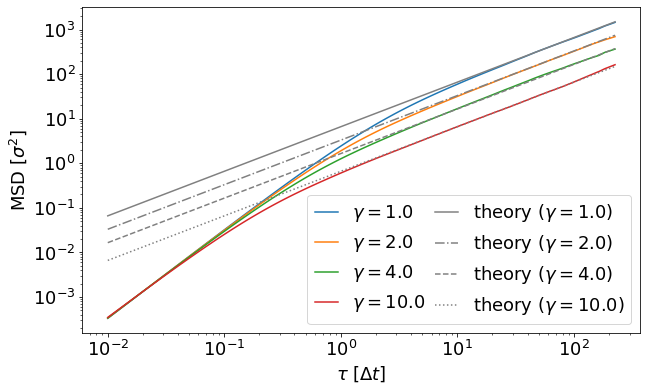### 3.2 Calculating the diffusion coefficient using the MSD¶

In this script an implicit solvent and a single particle are created and thermalized. The random forces on the particle will cause the particle to move. The mean squared displacement is calculated during the simulation via a multiple-tau correlator. Can you give an explanation for the quadratic time dependency for short times?

The MSD of a Brownian motion can be decomposed in three main regimes :

• for short lag times $\tau < \tau_\mathrm{p}$, the particle motion is not significantly impeded by solvent collisions: it's in the ballistic mode (collision-free regime) where $\operatorname{MSD}(t) \sim (k_\mathrm{B}T / \gamma) t^2$
• for long lag times $\tau > \tau_\mathrm{f}$, the particle motion is determined by numerous collisions with the solvent: it's in the diffusive mode where $\operatorname{MSD}(t) \sim 6t$
• for lag times between $\tau_\mathrm{p}$ and $\tau_\mathrm{f}$, there is a crossover mode

The values $\tau_\mathrm{p}$ and $\tau_\mathrm{f}$ can be obtained manually through visual inspection of the MSD plot, or more accurately by non-linear fitting .

Here, we are interested in the diffusion constant. Hence, we can ignore the ballistic regime and look at the diffusive regime in more detail.

Use the function curve_fit() from the module scipy.optimize to produce a fit for the linear regime and determine the diffusion coefficients for the different $\gamma$s.

For large $t$ the diffusion coefficient can be obtained using the fluctuation-dissipation theorem 

$$6D = \lim_{t\to\infty} \frac{\partial \operatorname{MSD}(t)}{\partial t},$$

where $D$ is straightforwardly given via the slope of the MSD in the diffusive mode.

Your results for the ($\gamma$-dependent) diffusivity coefficients should be saved in a Python-list diffusion_msd = [...].

In :
import scipy.optimize

def linear(x, a, b):
return a * x + b

# cutoffs for the diffusive regime (different for each gamma value)
tau_f_values = [24, 22, 20, 17]
# cutoff for the data series (larger lag times have larger variance due to undersampling)
cutoff_limit = 90

diffusion_msd = []

plt.figure(figsize=(10, 6))
plt.xlabel(r'$\tau$ [$\Delta t$]')
plt.ylabel(r'MSD [$\sigma^2$]')
for index, (tau_f, tau, msd) in enumerate(zip(tau_f_values, tau_results, msd_results)):
(a, b), _ = scipy.optimize.curve_fit(linear, tau[tau_f:cutoff_limit], msd[tau_f:cutoff_limit])
x = np.linspace(tau[tau_f], tau[cutoff_limit - 1], 50)
p = plt.plot(x, linear(x, a, b), '-')
plt.plot(tau[tau_f:cutoff_limit], msd[tau_f:cutoff_limit], 'o', color=p.get_color(),
label=fr'$\gamma=${gammas[index]:.1f}')
diffusion_msd.append(a / 6)

plt.legend()
plt.show()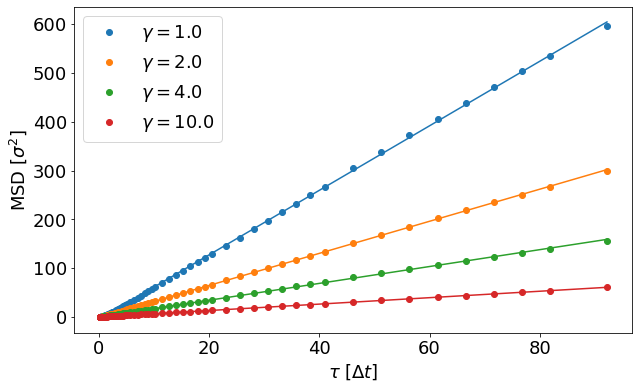### 3.3 Calculating the diffusion coefficient using the Green-Kubo relation¶

We now want to estimate the diffusion coefficient using the Green-Kubo relation given in Eq. (2). This approach is based on integrating the velocity-autocorrelation function, which should therefore be inspected first.

In :
plt.figure(figsize=(10, 6))
plt.xlabel(r"$\tau$ [$\Delta t$]")
plt.ylabel(r"$\langle {\bf v}(t_0) {\bf v}(t_0 + \tau) \rangle$")
plt.xlim([0.004, 2500])
plt.ylim([0.001, 5])
for index, (tau, vacf) in enumerate(zip(tau_results, vacf_results)):
plt.loglog(tau, vacf, label=fr'$\gamma={gammas[index]:.1f}$')
plt.legend()
plt.show()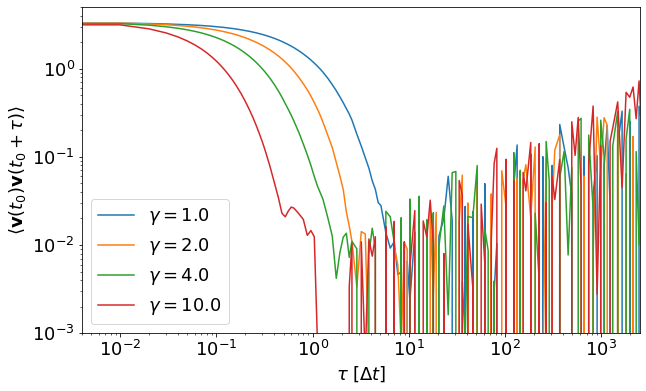We find that the velocity-autocorrelation function quickly decays towards zero. However, owing to the relatively short overall sampling time, only the first part of the correlation function is well-sampled and a lot of noise is found in the tail of the autocorrelation function already early on. The obvious solution would be to increase the sampling time and in a production setting one would definitely have to do so in order to smoothly resolve at least several relaxation times. However, depending on a system's characteristics, under certain conditions it might still be necessary to replace a noisy long-time tail with an analytical expression, fitted to the short-time part of the autocorrelation function (again over at least several decay times; typically one would smoothly transition between numerical short-time data and the analytical tail-fit).

A perfect smoothly sampled autocorrelation function could be integrated numerically, using e.g. numpy.trapz. Here, however, we will use the initial part of the velocity-autocorrelation function to obtain a fully analytic data representation. For a Brownian particle the velocity-autocorrelation is expected to follow a simple exponential decay.

Write a Python-function for the exponential decay. Fit your decay-function to the (short-time) correlation data and create a plot to visually verify that the analytic fits are indeed good representations of the data (the exponential decay should be a perfect match in the smooth region of the correlation function). You can copy and modify the plot script given above.

You should now estimate the $\gamma$-dependent diffusion coefficients using the analytically fitted data representations. That is, analytically integrate your decay-function from $0$ to $\infty$ and use this analytical integral and your fit parameters to calculate the diffusivity via the Green-Kubo expression given in Eq. (2). Save your results agin in a Python-list diffusion_gk = [...].

In :
def exp_decay(x, a, b):
return a * np.exp(-x / b)

diffusion_gk = []

linestyles = ["solid", "dashdot", "dashed", "dotted"]
plt.figure(figsize=(10, 6))
plt.xlabel(r"$\tau$ [$\Delta t$]")
plt.ylabel(r"$\langle {\bf v}(t_0) {\bf v}(t_0 + \tau) \rangle$")
plt.xlim([0.004, 2500])
plt.ylim([0.001, 5])
for index, (tau, vacf) in enumerate(zip(tau_results, vacf_results)):
plt.loglog(tau, vacf, label=fr"$\gamma=${gammas[index]:.1f}")
for index, (tau, vacf) in enumerate(zip(tau_results, vacf_results)):
(a, b), _ = scipy.optimize.curve_fit(exp_decay, tau[:60], vacf[:60])
xs = np.linspace(0.02, 100, 100000)
plt.loglog(xs, exp_decay(xs, a, b), linestyle=linestyles[index],
color="gray", label=fr"fit($\gamma=${gammas[index]:.1f})")
# Analytical calculation: int_0^infinity exp_decay(x, a, b) dx = a * b,
# consequently, the GK relation for the diffusivity is:
diffusion_gk.append(a * b / 3)
plt.legend(loc='upper right', ncol=2, columnspacing=0.5, handlelength=1.3, framealpha=1)
plt.show()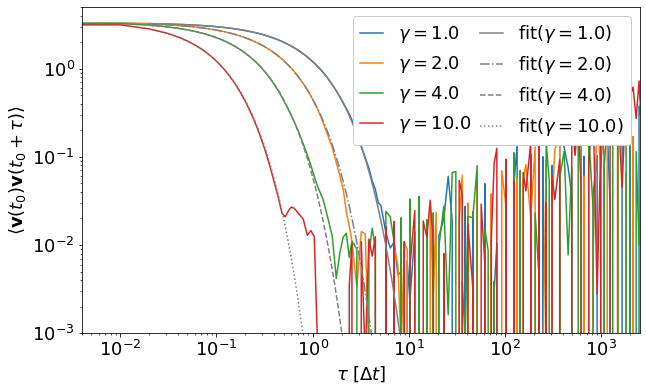### 3.3 Comparing to the Stokes-Einstein relation¶

Plot all diffusion coefficients (diffusion_msd, diffusion_gk) as a function of $\gamma$. What relation do you observe?

In the diffusive mode, one can derive $D = k_\mathrm{B}T / \gamma$ from the Stokes–Einstein relation . Compare your results to the Stokes-Einstein prediction.

In :
plt.figure(figsize=(10, 6))
plt.xlabel(r'$\gamma$')
plt.ylabel('Diffusion coefficient [$\sigma^2/t$]')
x = np.linspace(0.9 * min(gammas), 1.1 * max(gammas), 50)
y = KT / x
plt.plot(x, y, '-', label=r'$k_\mathrm{B}T\gamma^{-1}$')
plt.plot(gammas, diffusion_msd, 'o', label=r'$D_\mathrm{MSD}$')
plt.plot(gammas, diffusion_gk, '^', label=r'$D_\mathrm{GK}$')
plt.legend()
plt.show()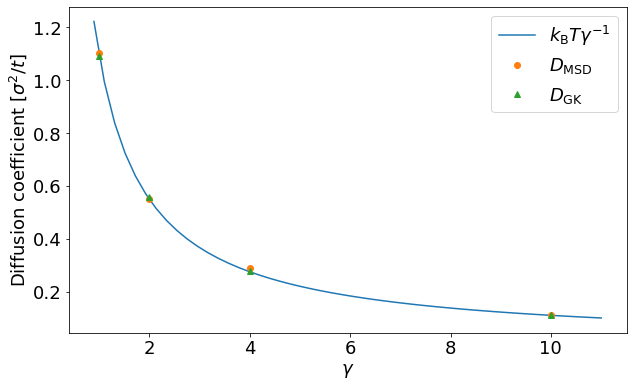R. Kubo. Statistical-Mechanical Theory of Irreversible Processes. I. General Theory and Simple Applications to Magnetic and Conduction Problems. J. Phys. Soc. Jpn., 12, 1957. doi:10.1143/JPSJ.12.570
 R. Huang, I. Chavez, K. Taute, et al. Direct observation of the full transition from ballistic to diffusive Brownian motion in a liquid. Nature Phys., 7, 2011. doi:10.1038/nphys1953
 M. K. Riahi, I. A. Qattan, J. Hassan, D. Homouz, Identifying short- and long-time modes of the mean-square displacement: An improved nonlinear fitting approach. AIP Advances, 9:055112, 2019. doi:10.1063/1.5098051
 R. Huang, I. Chavez, K. Taute, et al. Direct observation of the full transition from ballistic to diffusive Brownian motion in a liquid. Nature Phys., 7, 2011. doi:10.1038/nphys1953